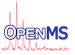OpenMS
AsymmetricStatistics< RealT > Class Template Reference

Internal class for asymmetric distributions. More...

#include <OpenMS/MATH/STATISTICS/AsymmetricStatistics.h>

Inheritance diagram for AsymmetricStatistics< RealT >:
[legend]
Collaboration diagram for AsymmetricStatistics< RealT >:
[legend]

## Public Member Functions

AsymmetricStatistics ()
Default constructor. More...

RealType variance1 () const
"variance to the left hand side" More...

RealType variance2 () const
"variance to the right hand side" More...

template<typename ProbabilityIterator , typename CoordinateIterator >
void update (ProbabilityIterator const probability_begin, ProbabilityIterator const probability_end, CoordinateIterator const coordinate_begin)
You can call this as often as you like, using different input vectors. More...Public Member Functions inherited from BasicStatistics< double >
BasicStatistics ()
Default constructor. More...

BasicStatistics (BasicStatistics const &arg)
Copy constructor. More...

BasicStatisticsoperator= (BasicStatistics const &arg)
Assignment. More...

void clear ()
Set sum, mean, and variance to zero. More...

void update (ProbabilityIterator probability_begin, ProbabilityIterator const probability_end)
This does the actual calculation. More...

void update (ProbabilityIterator const probability_begin, ProbabilityIterator const probability_end, CoordinateIterator const coordinate_begin)
This does the actual calculation. More...

RealType mean () const
Returns the mean. More...

void setMean (RealType const &mean)

RealType variance () const
Returns the variance. More...

void setVariance (RealType const &variance)

RealType sum () const
Returns the sum. More...

void setSum (RealType const &sum)

RealType normalDensity_sqrt2pi (RealType coordinate) const
Returns the density of the normal approximation at point, multiplied by sqrt( 2 * pi ). This saves a division operation compared to normalDensity() More...

RealType normalDensity (RealType const coordinate) const
See normalDensity_sqrt2pi(). Returns the density of the normal distribution at point. More...

void normalApproximation (probability_container &probability)
The argument probability is filled with values according to the normal approximation. Its size() is not changed. The approximation takes place at coordinate positions 0, 1, ..., size()-1. More...

void normalApproximation (probability_container &probability, typename probability_container::size_type const size)

void normalApproximation (probability_container &probability, coordinate_container const &coordinate)
The argument probability is filled with values according to the normal approximation. The second argument coordinate contains the positions where the approximation takes place. probability.size() is set to coordinate.size(). More...

## Protected Attributes

Protected Members
RealType variance1_

RealType variance2_Protected Attributes inherited from BasicStatistics< double >
RealType mean_

RealType variance_

RealType sum_

## Private Types

typedef BasicStatistics< RealT > Base
The real type and basic statistics specified as template argument. More...

typedef Base::RealType RealType

## Private Member Functions

void clear ()
Set sum, mean, and variance to zero. More...

## Private Attributes

RealType sum_

RealType mean_

RealType variance_Public Types inherited from BasicStatistics< double >
typedef double RealType
The real type specified as template argument. More...

typedef std::vector< RealTypeprobability_container

typedef std::vector< RealTypecoordinate_containerStatic Public Member Functions inherited from BasicStatistics< double >
static RealType sqrt2pi ()
Returns sqrt( 2 * pi ), which is useful to normalize the result of normalDensity_sqrt2pi(). More...

## Detailed Description

### template<typename RealT = double> class OpenMS::Math::AsymmetricStatistics< RealT >

Internal class for asymmetric distributions.

Internal class for asymmetric distributions used for consistency with BasisStatistic class

## ◆ Base

 typedef BasicStatistics Base
private

The real type and basic statistics specified as template argument.

## ◆ RealType

 typedef Base::RealType RealType
private

## ◆ AsymmetricStatistics()

 AsymmetricStatistics ( )
inline

Default constructor.

## ◆ clear()

 void clear
inlineprivate

Set sum, mean, and variance to zero.

## ◆ update()

 void update ( ProbabilityIterator const probability_begin, ProbabilityIterator const probability_end, CoordinateIterator const coordinate_begin )
inline

You can call this as often as you like, using different input vectors.

## ◆ variance1()

 RealType variance1 ( ) const
inline

"variance to the left hand side"

References AsymmetricStatistics< RealT >::variance1_.

## ◆ variance2()

 RealType variance2 ( ) const
inline

"variance to the right hand side"

References AsymmetricStatistics< RealT >::variance2_.

## ◆ mean_

 RealType mean_
private

## ◆ sum_

 RealType sum_
private

## ◆ variance1_

 RealType variance1_
protected

## ◆ variance2_

 RealType variance2_
protected

## ◆ variance_

 RealType variance_
private